# Self-counting machine

The self-counting machine works exactly like a calculator. The innkeeper wanted to add several three-digit natural numbers on his own. On the first attempt, he got the result in 2224. To check, he added these numbers again and he got 2198. Therefore, he added these numbers again and now he got the sum of 2204. He always entered the fifth added number incorrectly, because he did not press any of its digits hard enough in each attempt, and in the self-calculation, he always entered a two-digit number instead of a three-digit number. He did not make any other errors in the census, and this time the self-count also worked flawlessly. What is the correct sum of inn numbers?

s =  2324

### Step-by-step explanation: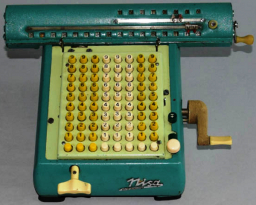Did you find an error or inaccuracy? Feel free to write us. Thank you!Tips to related online calculators
Do you have a system of equations and looking for calculator system of linear equations?
Do you solve Diofant problems and looking for a calculator of Diofant integer equations?

## Related math problems and questions:

• All pairs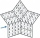Determine all pairs (m, n) of natural numbers for which is true: m s (n) = n s (m) = 70, where s (a) denotes the digit sum of the natural number a.
• Z9-I-4Kate thought a five-digit integer. She wrote the sum of this number and its half at the first line to the workbook. On the second line wrote a total of this number and its one fifth. On the third row, she wrote a sum of this number and its one nines. Fina
• Five-digit numberAnna thinks of a five-digit number that is not divisible by three or four. If he increments each digit by one, it gets a five-digit number that is divisible by three. If he reduces each digit by one, he gets a five-digit number divisible by four. If it sw
• Phone number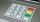Ivan's phone number ends with a four-digit number: When we subtract the first from the fourth digit of this four-digit number, we get the same number as when we subtract the second from the third digit. If we write the four-digit number from the back and
• Digit sumThe digit sum of the two-digit number is nine. When we turn figures and multiply by the original two-digit number, we get the number 2430. What is the original two-digit number?
• Four-digit numbers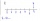Find four-digit numbers where all the digits are different. For numbers, the sum of the third and fourth digits is twice the sum of the first two digits, and the sum of the first and fourth digits is equal to the sum of the second and third digits. The di
• Octahedron - sumOn each wall of a regular octahedron is written one of the numbers 1, 2, 3, 4, 5, 6, 7 and 8, wherein on different sides are different numbers. For each wall John make the sum of the numbers written of three adjacent walls. Thus got eight sums, which also
• Two-digit numberIn a two-digit number, the number of tens is three greater than the number of units. If we multiply the original number by a number written in the same digits but in reverse order, we get product 3 478. Find the original number.
• Alarm clockThe old watchmaker has a unique digital alarm in its collection that rings whenever the sum of digits of the alarm is equal to 21. Find out when the alarm clock will ring. What is their number? List all options . ..
• Last digitWhat is the last number of 2016 power of 2017
• David numberJana and David train the addition of the decimal numbers so that each of them will write a single number and these two numbers then add up. The last example was 11.11. David's number had the same number of digits before the decimal point, the Jane's numbe
• Digits A, B, CFor the various digits A, B, C is true: the square root of the BC is equal to the A and sum B+C is equal to A. Calculate A + 2B + 3C. (BC is a two-digit number, not a product).
• Phone numbersHow many 7-digit telephone numbers can be compiled from the digits 0,1,2,..,8,9 that no digit is repeated?
• MO Z8-I-1 2018Fero and David meet daily in the elevator. One morning they found that if they multiply their current age, they get 238. If they did the same after four years, this product would be 378. Determine the sum of the current ages of Fero and David.
• One million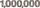Write the million number (1000000) using only nine numbers and algebraic operations plus, minus, times, divided, powers, and squares. Find at least three different solutions.
• Star equationWrite digits instead of stars so that the sum of the written digits is odd and is true equality: 42 · ∗8 = 2 ∗∗∗
• A five-digit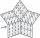A five-digit odd number has the sum of all digits (digits) five and contains two zeros. If we move each digit in the number one place to the left and move the first digit to the last place, we get a number 20,988 smaller. Find this unknown five-digit numb